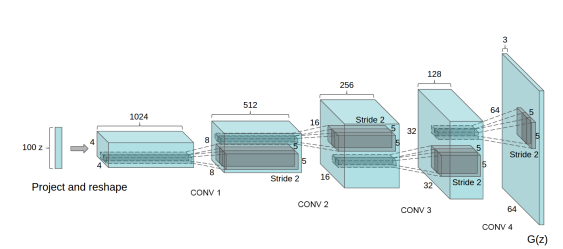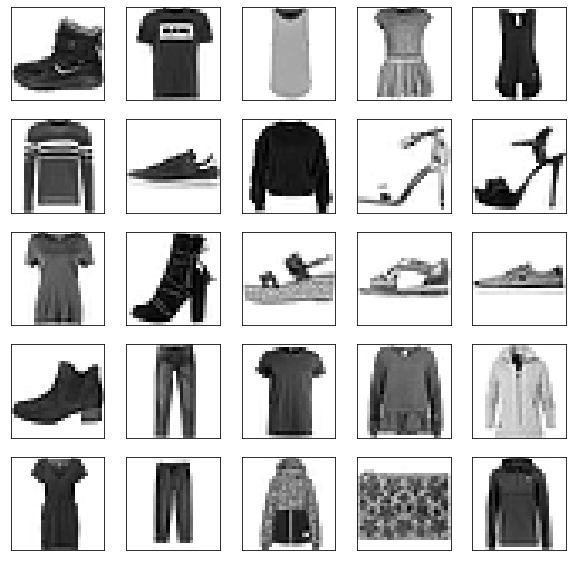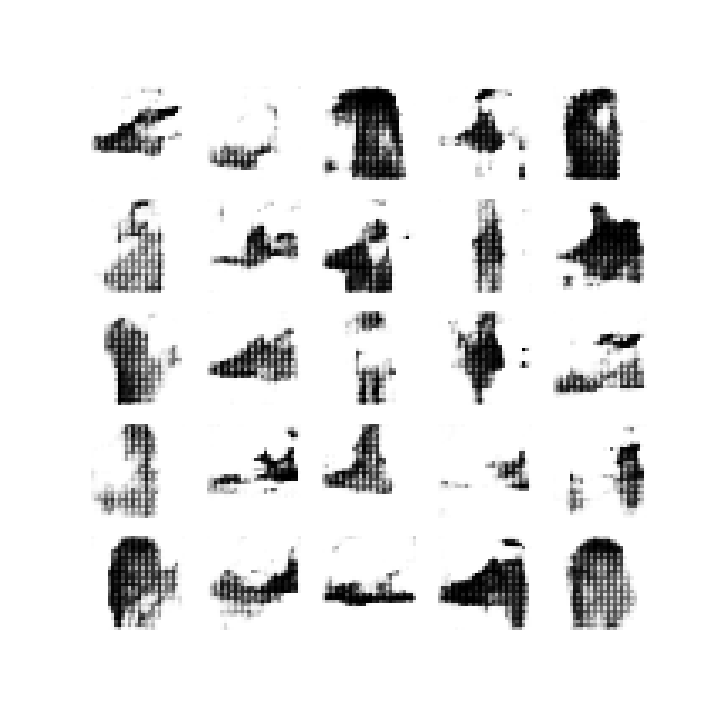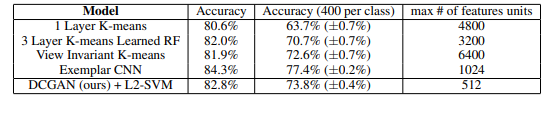# Deep Convolutional GAN with Keras

Deep Convolutional GAN (DCGAN) was proposed by a researcher from MIT and Facebook AI research .It is widely used in many convolution based generation based techniques. The focus of this paper was to make training GANs stable . Hence, they proposed some architectural changes in computer vision problem. In this article we will be using DCGAN on fashion MNIST dataset to generate the images related to clothes.

Architecture:The generator of the DCGAN architecture takes 100 uniform generated values using normal distribution as an input. First, it changes the dimension  to 4x4x1024 and performed a fractionally strided convolution in 4 times with stride of 1/2 (this means every time when applied, it doubles the image dimension while reducing the number of output channels). The generated output has dimensions of (64, 64, 3). There are some architectural changes proposed in generator such as removal of all fully connected layer, use of Batch Normalization which helps in stabilizing training.  In this paper, the authors use ReLU activation function in all layers of generator, except for the output layers. We will be implementing generator with similar guidelines but not completely same architecture.

The role of the discriminator here is to determine that the image comes from either real dataset or generator.   The discriminator can be simply designed similar to a convolution neural network that performs a image classification task. However, the authors of this paper suggested some changes in the discriminator architecture. Instead of fully connected layers, they used only strided-convolutions with LeakyReLU as activation function, the input of the generator is a single image from dataset or generated image and the output is a score that determines the image is real or generated.

Implementation:

In this section we will be discussing implementation of DCGAN in keras, since our dataset in Fashion MNIST dataset, this dataset contains images of  size (28, 28) of 1 color channel instead of (64, 64) of 3 color channels. So, we needs to make some  changes in the architecture  , we  will be discussing these  changes as we go along.

• In first step, we need to  import the necessary classes such as TensorFlow, keras  , matplotlib etc. We will be using TensorFlow version 2.  This version of tensorflow provides inbuilt support for Keras library as its default High level API.

 `# code % matplotlib inline ` `import` `tensorflow as tf ` `from` `tensorflow ``import` `keras ` `import` `numpy as np ` `import` `matplotlib.pyplot as plt ` `from` `tqdm ``import` `tqdm ` `from` `IPython ``import` `display ` ` `  `# Check tensorflow version ` `print``(``'Tensorflow version:'``, tf.__version__) `

• Now we load the fashion-MNIST dataset, the good thing is that dataset can be imported  from tf.keras.datasets API. So, we don’t need to load datasets manually by copying files. This dateset contains 60k training images and 10k test images each of dimensions(28, 28, 1). Since the value of each pixel is in the range (0, 255), we divide these values by 255 to normalize it.

 `(x_train, y_train), (x_test, y_test) ``=` `tf.keras.datasets.fashion_mnist.load_data() ` `x_train ``=` `x_train.astype(np.float32) ``/` `255.0` `x_test ``=` `x_test.astype(np.float32) ``/` `255.0` `x_train.shape, x_test.shape `

`((60000, 28, 28), (10000, 28, 28))`
• Now in the next step, we will  be visualizing some of the images from Fashion-MNIST dateset, we use matplotlib library for that.

 `# We plot first 25 images of training dataset ` `plt.figure(figsize ``=``(``10``, ``10``)) ` `for` `i ``in` `range``(``25``): ` `    ``plt.subplot(``5``, ``5``, i ``+` `1``) ` `    ``plt.xticks([]) ` `    ``plt.yticks([]) ` `    ``plt.grid(``False``) ` `    ``plt.imshow(x_train[i], cmap ``=` `plt.cm.binary) ` `plt.show() `Original Fashion MNIST images

• Now, we define training parameters such as batch size and divides the dataset into batch size and fills those batch size by randomly sampling the training data.

 `# code ` `batch_size ``=` `32` `# This dataset fills a buffer with buffer_size elements, ` `# then randomly samples elements from this buffer,  ` `# replacing the selected elements with new elements. ` `def` `create_batch(x_train): ` `  ``dataset ``=` `tf.data.Dataset.from_tensor_slices(x_train).shuffle(``1000``) ` `  ``# Combines consecutive elements of this dataset into batches. ` ` `  `  ``dataset ``=` `dataset.batch(batch_size, drop_remainder ``=` `True``).prefetch(``1``) ` `  ``# Creates a Dataset that prefetches elements from this dataset ` `  ``return` `dataset `

• Now, we define the generator architecture, this generator architecture takes a vector of size 100 and first reshape that into (7, 7, 128) vector then applied transpose convolution in combination with batch normalization. The output of this generator is a trained an image of dimension (28, 28, 1).

 `# code ` `num_features ``=` `100` ` `  `generator ``=` `keras.models.Sequential([ ` `    ``keras.layers.Dense(``7` `*` `7` `*` `128``, input_shape ``=``[num_features]), ` `    ``keras.layers.Reshape([``7``, ``7``, ``128``]), ` `    ``keras.layers.BatchNormalization(), ` `    ``keras.layers.Conv2DTranspose( ` `        ``64``, (``5``, ``5``), (``2``, ``2``), padding ``=``"same"``, activation ``=``"selu"``), ` `    ``keras.layers.BatchNormalization(), ` `    ``keras.layers.Conv2DTranspose( ` `        ``1``, (``5``, ``5``), (``2``, ``2``), padding ``=``"same"``, activation ``=``"tanh"``), ` `]) ` `generator.summary() `

```Model: "sequential"
_________________________________________________________________
Layer (type)                 Output Shape              Param #
=================================================================
dense (Dense)                (None, 6272)              633472
_________________________________________________________________
reshape (Reshape)            (None, 7, 7, 128)         0
_________________________________________________________________
batch_normalization (BatchNo (None, 7, 7, 128)         512
_________________________________________________________________
conv2d_transpose (Conv2DTran (None, 14, 14, 64)        204864
_________________________________________________________________
batch_normalization_1 (Batch (None, 14, 14, 64)        256
_________________________________________________________________
conv2d_transpose_1 (Conv2DTr (None, 28, 28, 1)         1601
=================================================================
Total params: 840, 705
Trainable params: 840, 321
Non-trainable params: 384
_________________________________________________________________```
• Now, we define out discriminator architecture, the discriminator takes image of size  28*28 with 1 color channel and output a scalar value representing image from either dataset or generated image.

 `discriminator ``=` `keras.models.Sequential([ ` `    ``keras.layers.Conv2D(``64``, (``5``, ``5``), (``2``, ``2``), padding ``=``"same"``, input_shape ``=``[``28``, ``28``, ``1``]), ` `    ``keras.layers.LeakyReLU(``0.2``), ` `    ``keras.layers.Dropout(``0.3``), ` `    ``keras.layers.Conv2D(``128``, (``5``, ``5``), (``2``, ``2``), padding ``=``"same"``), ` `    ``keras.layers.LeakyReLU(``0.2``), ` `    ``keras.layers.Dropout(``0.3``), ` `    ``keras.layers.Flatten(), ` `    ``keras.layers.Dense(``1``, activation ``=``'sigmoid'``) ` `]) ` `discriminator.summary()`

```Model: "sequential_1"
_________________________________________________________________
Layer (type)                 Output Shape              Param #
=================================================================
conv2d (Conv2D)              (None, 14, 14, 64)        1664
_________________________________________________________________
leaky_re_lu (LeakyReLU)      (None, 14, 14, 64)        0
_________________________________________________________________
dropout (Dropout)            (None, 14, 14, 64)        0
_________________________________________________________________
conv2d_1 (Conv2D)            (None, 7, 7, 128)         204928
_________________________________________________________________
leaky_re_lu_1 (LeakyReLU)    (None, 7, 7, 128)         0
_________________________________________________________________
dropout_1 (Dropout)          (None, 7, 7, 128)         0
_________________________________________________________________
flatten (Flatten)            (None, 6272)              0
_________________________________________________________________
dense_1 (Dense)              (None, 1)                 6273
=================================================================
Total params: 212, 865
Trainable params: 212, 865
Non-trainable params: 0
_________________________________________________________________```
•  Now we need to compile the our DCGAN model (combination of generator and discriminator), we will first compile discriminator and set its  training to False, because we first want to train the generator.

 `# compile discriminator using binary cross entropy loss and adam optimizer ` `discriminator.``compile``(loss ``=``"binary_crossentropy"``, optimizer ``=``"adam"``) ` `# make  discriminator no-trainable as of  now ` `discriminator.trainable ``=` `False` `# Combine both generator and discriminator ` `gan ``=` `keras.models.Sequential([generator, discriminator]) ` `# compile generator using binary cross entropy loss and adam optimizer ` ` `  `gan.``compile``(loss ``=``"binary_crossentropy"``, optimizer ``=``"adam"``)`

•   Now, we define the training procedure for this GAN model, we will be using tqdm package which we have imported earlier., this package help in visualizing training.

 `seed ``=` `tf.random.normal(shape ``=``[batch_size, ``100``]) ` ` `  `def` `train_dcgan(gan, dataset, batch_size, num_features, epochs ``=` `5``): ` `    ``generator, discriminator ``=` `gan.layers ` `    ``for` `epoch ``in` `tqdm(``range``(epochs)): ` `        ``print``() ` `        ``print``(``"Epoch {}/{}"``.``format``(epoch ``+` `1``, epochs)) ` ` `  `        ``for` `X_batch ``in` `dataset: ` `            ``# create a random noise of sizebatch_size * 100 ` `            ``# to passit into the generator ` `            ``noise ``=` `tf.random.normal(shape ``=``[batch_size, num_features]) ` `            ``generated_images ``=` `generator(noise) ` ` `  `            ``# take batch of generated image and real image and ` `            ``#  use them to train  the discriminator ` `            ``X_fake_and_real ``=` `tf.concat([generated_images, X_batch], axis ``=` `0``) ` `            ``y1 ``=` `tf.constant([[``0.``]] ``*` `batch_size ``+` `[[``1.``]] ``*` `batch_size) ` `            ``discriminator.trainable ``=` `True` `            ``discriminator.train_on_batch(X_fake_and_real, y1) ` ` `  `            ``# Here we will be training our GAN model, in this step ` `            ``#  we pass noise that uses geeneratortogenerate the image ` `            ``#  and pass it with labels as  So, it can fool the discriminatoe ` `            ``noise ``=` `tf.random.normal(shape ``=``[batch_size, num_features]) ` `            ``y2 ``=` `tf.constant([[``1.``]] ``*` `batch_size) ` `            ``discriminator.trainable ``=` `False` `            ``gan.train_on_batch(noise, y2) ` ` `  `            ``# generate images for the GIF as we go ` `            ``generate_and_save_images(generator, epoch ``+` `1``, seed) ` ` `  `    ``generate_and_save_images(generator, epochs, seed) `

• Now we define a function that generate and save images from generator (during training). We will use these generated images to plot the GIF later.

 `# code ` `def` `generate_and_save_images(model, epoch, test_input): ` `  ``predictions ``=` `model(test_input, training ``=` `False``) ` ` `  `  ``fig ``=` `plt.figure(figsize ``=``(``10``, ``10``)) ` ` `  `  ``for` `i ``in` `range``(``25``): ` `      ``plt.subplot(``5``, ``5``, i ``+` `1``) ` `      ``plt.imshow(predictions[i, :, :, ``0``] ``*` `127.5` `+` `127.5``, cmap ``=``'binary'``) ` `      ``plt.axis(``'off'``) ` ` `  `  ``plt.savefig(``'image_epoch_{:04d}.png'``.``format``(epoch)) `

• Now, we need to train the model but before that we also need to create batches of training data and add a dimension that represents number  of color maps.

 `# reshape to add a color map ` `x_train_dcgan ``=` `x_train.reshape(``-``1``, ``28``, ``28``, ``1``) ``*` `2.` `-` `1.` `# create batches ` `dataset ``=` `create_batch(x_train_dcgan) ` `# callthe training function with 10 epochs and record time %% time ` `train_dcgan(gan, dataset, batch_size, num_features, epochs ``=` `10``) `

```0%|          | 0/10 [00:00<?, ?it/s]
Epoch 1/10

10%|?         | 1/10 [01:04<09:39, 64.37s/it]
Epoch 2/10

20%|??        | 2/10 [02:10<08:39, 64.99s/it]
Epoch 3/10

30%|???       | 3/10 [03:14<07:33, 64.74s/it]
Epoch 4/10

40%|????      | 4/10 [04:19<06:27, 64.62s/it]
Epoch 5/10

50%|?????     | 5/10 [05:23<05:22, 64.58s/it]
Epoch 6/10

60%|??????    | 6/10 [06:27<04:17, 64.47s/it]
Epoch 7/10

70%|???????   | 7/10 [07:32<03:13, 64.55s/it]
Epoch 8/10

80%|????????  | 8/10 [08:37<02:08, 64.48s/it]
Epoch 9/10

90%|????????? | 9/10 [09:41<01:04, 64.54s/it]
Epoch 10/10

100%|??????????| 10/10 [10:46<00:00, 64.61s/it]
CPU times: user 7min 4s, sys: 33.3 s, total: 7min 37s
Wall time: 10min 46s```
• Now we will  define a function that takes  the save images and convert into GIF. We use this function from here

 `import` `imageio ` `import` `glob ` ` `  `anim_file ``=` `'dcgan_results.gif'` ` `  `with imageio.get_writer(anim_file, mode ``=``'I'``) as writer: ` `  ``filenames ``=` `glob.glob(``'image*.png'``) ` `  ``filenames ``=` `sorted``(filenames) ` `  ``last ``=` `-``1` `  ``for` `i, filename ``in` `enumerate``(filenames): ` `    ``frame ``=` `2``*``(i) ` `    ``if` `round``(frame) > ``round``(last): ` `      ``last ``=` `frame ` `    ``else``: ` `      ``continue` `    ``image ``=` `imageio.imread(filename) ` `    ``writer.append_data(image) ` `  ``image ``=` `imageio.imread(filename) ` `  ``writer.append_data(image) ` `display.Image(filename ``=` `anim_file) `Generated Images results

Results and Conclusion:

• To evaluate the quality of the representations learned by DCGANs for supervised tasks, the authors train the model on ImageNet-1k and then use the discriminator’s convolution features from all layers, max pooling each layers representation to produce a 4 × 4 spatial grid. These features are then flattened and concatenated to form a 28672 dimensional vector and a regularized linear L2-SVM classifier is trained on top of them.  This model is then evaluated on CIFAR-10 dataset but not trained don it. The model reported an accuracy of 82 % which also displays robustness of the model.• On Street View Housing Number dataset, it achieved a validation loss of 22% which is the new state-of-the-art, even discriminator architecture when supervise trained as a CNN model has more validation loss than it.

References:

My Personal Notes arrow_drop_upCheck out this Author's contributed articles.

If you like GeeksforGeeks and would like to contribute, you can also write an article using contribute.geeksforgeeks.org or mail your article to contribute@geeksforgeeks.org. See your article appearing on the GeeksforGeeks main page and help other Geeks.

Please Improve this article if you find anything incorrect by clicking on the "Improve Article" button below.

Article Tags :
Practice Tags :

Be the First to upvote.

Please write to us at contribute@geeksforgeeks.org to report any issue with the above content.